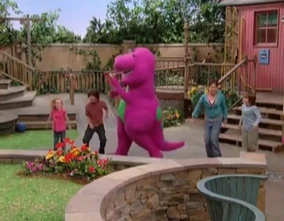## FANDOM

2,540 Pages

 Barney Songs Let's GoDebuted in Let's Go to the Fire House Written by Scott Erickson Usage 2007-2010 previousThe Dance of the Dragon nextLiving in the Fire House
"Let's Go" is an original Barney song that first appeared in Let's Go to the Fire House. It is not to be confused with "Let's Go On An Adventure" or "The Adventure Song".

## Lyrics

Let's go! (Let's go!)
Let's go! (Let's go!)
We can hardly wait
Let's go! (Let's go!)
It's going to be so great.
Let's go, let's go,
let's go, let's go!

Let's go and
explore the world
Each day will bring something new
Anything is possible,
Make a wish and it might come true.

Let's go! (Let's go!)
Let's go! (Let's go!)
We can hardly wait
Let's go! (Let's go!)
It's going to be so great.
Let's go, let's go,
let's go, let's go!

Let's go! (Let's go!)
Let's Go! (Let's go!)
We can hardly wait
Let's go! (Let's go!)
It's going to be so great.
Let's go, let's go,
let's go, let's go!

Let's go, let's go,
let's go, let's go!

(Let's go!)
(Let's go!)
(Let's go!)
(Let's go, let's go, let's go, let's go)

Let's go to the fire house to see what happened there
Is there a big red truck like in my book?
To fight a fire what do they where?

Let's go! (Let's go!)
Let's Go! (Let's go!)
We can hardly wait
Let's go! (Let's go!)
It's going to be so great.
Let's go, let's go,
let's go, let's go!

Let's go and discover how the firefighters get prepared
When hear the sirens and the bells do you think they ever get scared?

Let's go! (Let's go!)
Let's go! (Let's go!)
It's gonna be so great!
Let's go! (Let's go!)
We can hardly wait
Let's go, let's go,
let's go, let's go!

Let's go! (Let's go!)
Let's Go! (Let's go!)
It's going to be so great
Let's go! (Let's go!)
We can hardly wait
Let's go, let's go,
let's go, let's go!

Let's go, let's go,
let's go, let's go!

## Barney Song Used In...

Community content is available under CC-BY-SA unless otherwise noted.